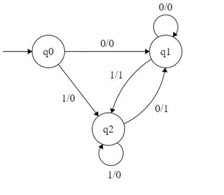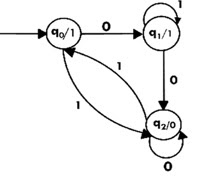# Differentiate between Mealy machine and Moore machine in TOC

## Mealy Machine

In a Mealy machine the output symbol depends upon the present input symbol and present state of the machine.

In the Mealy machine, the output is represented with each input symbol and each state is separated by /.

The Mealy machine can be described by six tuples (Q, q0, Σ, O, δ, λ')

Where,

• Q: Finite set of states.
• q0: Initial state of machine.
• Σ: Finite set of input alphabet.
• O: Output alphabet.
• δ: Transition function where Q × Σ → Q.
• λ': Output function where Q × Σ → O.

In the Mealy machine, the output is represented with each input symbol and each state is separated by /.

The length of output for a mealy machine is equal to the length of input.

## Moore Machine

Moore machine is a finite state machine in which the next state is decided by the current state and current input symbol.

The output symbol at a given time depends only on the present state of the machine.

Moore machine has six tuples (Q, q0, Σ, O, δ, λ)

Where,

• Q: Finite set of states.
• q0: Initial state of machine.
• Σ: Finite set of input symbols.
• O: Output alphabet.
• δ: Transition function where Q × Σ → Q.
• λ': Output function where Q → O.

## Differences

The major differences between Mealy machine and Moore machine are as follows −

Mealy MachineMoore Machine
Output depends on present state as well as present input.Output depends only upon the present state.
If input changes, output also changes.If input changes, output does not change.
Less number of states are required.More states are required.
Asynchronous output generation.Synchronous output and state generation.
Output is placed on transition.Output is placed on state.
It is difficult to design.Easy to design.